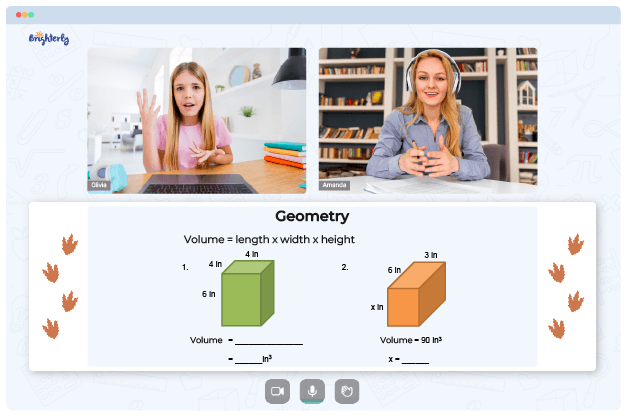# Perimeter Worksheets

Even though area and perimeter usually go together, kids might get confused if you don’t clearly differentiate between the two. Remember that area and perimeter are two different concepts, so it would help if you teach one concept before moving on to another. If you choose to teach kids perimeter, try to offer perimeter worksheets to kids for practicing.

## Free Perimeter Worksheets from Brighterly

Leave your email and we will send you worksheets

worksheets sent successfully

Finding perimeter worksheets does not have to be difficult if you know where to search. Worksheets show that a two-dimensional shape’s area measures how much room there is within the shape’s perimeter. Tutors use a perimeter worksheet to explain to kids that to get a shape’s perimeter, they need to sum the lengths of all sides.

Students who continuously use free perimeter worksheets will remember that a perimeter is the length of a shape’s edge, while an area is the space a shape covers.

Math for Kids

Is Your Child Struggling With Math?
1:1 Online Math TutoringBrighterly is an online math learning platform whose tutors help students scale through learning hurdles. Tutors understand that learning has to be a smooth and pleasant process, so they use perimeter worksheets in PDF files. Worksheets usually come with step-by-step instructions to help kids grasp information easily.

## How Perimeter Worksheets Can Make Learning Exciting

Perimeter is an essential mathematical topic a kid learns while studying shapes and measurement. At school, students learn how to calculate a perimeter of a 2-dimensional object.

Helping your kid with online math lessons for 4th grade. Expert tutors inspiring kids to love math so they can excel at It.

They can use measure perimeter worksheets to do fun, exciting activities like measuring a school playground or their home’s driveway.

The ability to measure a perimeter is a practical life skill, so every kid should know how to solve perimeter questions in math.

Leave your email and we will send you worksheets

worksheets sent successfully### Perimeter Worksheet PDF

Perimeter Worksheet### Perimeter Worksheet PDF

Perimeter Worksheets PDF### Perimeter Worksheet PDF

Finding Perimeter Worksheets### Perimeter Worksheet PDF

Free Perimeter Worksheets

The use of perimeter word problems worksheets in PDF files allows students to determine the length of unlabeled edges given the shape’s perimeter easily. The tools also make teaching faster for math tutors, especially for those managing a large class. Exercises in worksheets begin from the basics, so kids can visualize learning using simple examples such as walking around a field or erecting a fence.

### Area and Perimeter Worksheets

Need help with Geometry?• Does your child struggle to grasp geometry concepts?
• Try learning with an online tutor.

Is your child having difficulties with understanding of geometry? An online tutor could provide the necessary support.